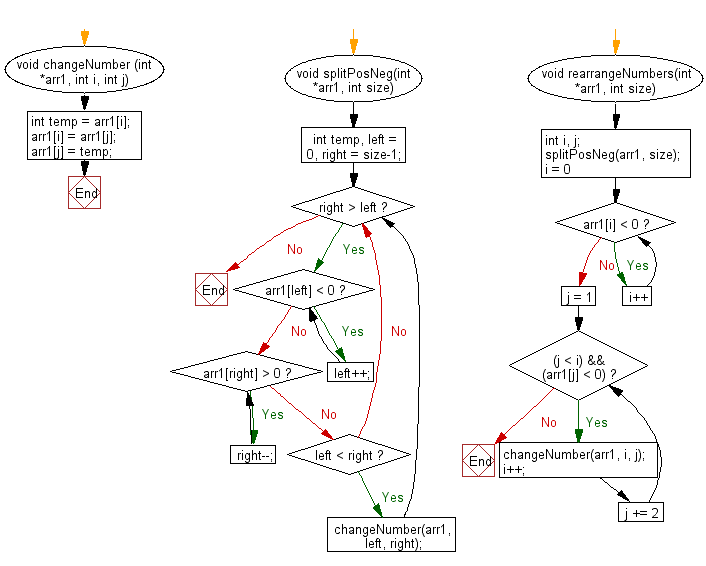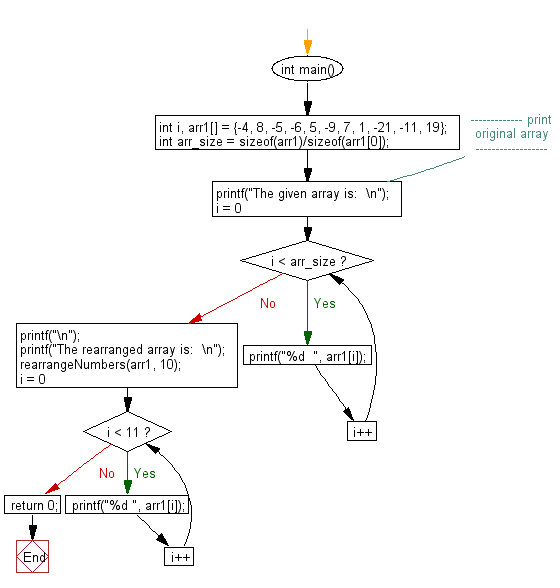﻿ C exercises: Rearrange positive and negative numbers alternatively in a given array - w3resource# C Exercises: Rearrange positive and negative numbers alternatively in a given array

## C Array: Exercise-93 with Solution

Write a program in C to rearrange positive and negative numbers alternatively in a given array.

N.B.: If positive numbers are more they appear at the end and for also negative numbers, they too appear in the end of the array.

Sample Solution:

C Code:

``````#include <stdio.h>

void changeNumber (int *arr1, int i, int j)
{
int temp = arr1[i];
arr1[i] = arr1[j];
arr1[j] = temp;
}

void splitPosNeg(int *arr1, int size)
{
int temp, left = 0, right = size-1;
while(right > left)
{
while(arr1[left] < 0)
left++;
while(arr1[right] > 0)
right--;
if(left < right)
{
changeNumber(arr1, left, right);
}
}
}

void rearrangeNumbers(int *arr1, int size)
{
int i, j;
splitPosNeg(arr1, size);
for(i = 0; arr1[i] < 0; i++);
for(j = 1; (j < i) && (arr1[j] < 0); j += 2)
{
changeNumber(arr1, i, j);
i++;
}
return;
}

int main()
{
int i, arr1[] = {-4, 8, -5, -6, 5, -9, 7, 1, -21, -11, 19};
int arr_size = sizeof(arr1)/sizeof(arr1);
//------------- print original array ------------------
printf("The given array is:  \n");
for(i = 0; i < arr_size; i++)
{
printf("%d  ", arr1[i]);
}
printf("\n");
//-----------------------------------------------------------
printf("The rearranged array is:  \n");
rearrangeNumbers(arr1, 10);
for(i = 0; i < 11; i++){
printf("%d ", arr1[i]);
}
return 0;
}
```
```

Sample Output:

```The given array is:
-4  8  -5  -6  5  -9  7  1  -21  -11  19
The rearranged array is:
-4 7 -5 1 -21 5 -11 8 -9 19 -6
```

Flowchart:C Programming Code Editor:

Improve this sample solution and post your code through Disqus.

﻿